# Pocket Pairs: I

Brian Alspach

Poker Digest Vol. 5, No. 1, December 28-January 10, 2002

Every now and then in hold'em, a table is treated to two players who've been dealt the same pocket pair. Determining the probability of this happening is an easy application of inclusion-exclusion. Let's go through the steps outlining the method but excluding the details.

Since we are interested only in the outcome of two players being dealt the same pocket pair and not which players are dealt the pairs, we are discussing player semideals''. The number of player semideals in a 10-handed game is easy to determine. There are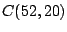ways of choosing the 20 cards for the players to receive. Once the 20 cards are chosen, there are 19!! ways of breaking the 20 cards into 10 hands of two cards each. Recall that.

The product ofand 19!! is then the number of player semideals. This product is 82,492,346,176,096,206,475,125 and is the last huge number I'll throw at you in this article.

In order to get the exact probability, and the reason we use inclusion-exclusion, we have to take into account the fact there could be more than two people having the same pocket pair as someone else. In fact, it is possible (though highly unlikely) that five sets of identical pairs have been dealt. Let's count how many ways this could happen.

There arechoices for the ranks. Each set of four cards may be split into two hands in three ways. Thus, we multiplyby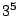and obtain 312,741 player-semideals with five sets of identical pairs. Use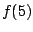to denote 312,741. The probability of such a player-semideal has 17 zeros following the decimal point. I said it was highly unlikely.

We do one more example to illustrate the procedure.

Let's determine how many player semideals have exactly four sets of identical pairs. There are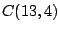choices for the ranks of the pairs; there are three ways to split the four cards of each rank into two hands; there arechoices for the remaining four cards; and there are three choices for splitting the four remaining cards into two hands. Multiplying all these numbers gives us the number of player semideals with at least four sets of identical pairs.

However, any player semideal counted inis counted five times in the preceding product. Thus, we subtract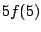and obtain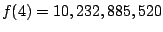player semideals with precisely four sets of identical pairs.

We determine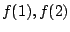and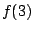in the same way. We then take the sum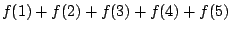to obtain the number of player semideals with at least two people being dealt pairs of the same rank. We divide by the total number of player semideals given above and obtain a probability of .00215899 that, upon randomly dealing two cards to each of ten players, there is at least one set of pairs of the same rank dealt to two players. This is about once in every 463 deals.

The preceding numbers have no restriction on the rank of the pairs. What causes the most drama at the table is when two players both are dealt pocket aces or pocker kings. So let's work out the probability that two players are dealt pocket aces.

Here we can simply use proportionality arguments. The hardest work is obtaining the numbers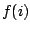, but once they have been obtained we can use them as follows. Now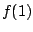is the number of player semideals with precisely one rank for which two players are dealt pairs of that rank. Since each rank occurs equally often, we divideby 13 to obtain the number of player semideals with two players being dealt pocket aces and no other rank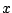with two players being dealt pairs of rank.

Continuing,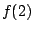is the number of player semideals with exactly two ranks for which four players have pairs of these two ranks. There are 78 combinations of two ranks chosen from 13, and there are 12 combinations of ranks including ace. That is, 2/13 of the combinations involve ace. So we multiplyby 2/13 to get those which involve two players with pocket aces.

Similarly, we multiplyby 3/13,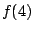by 4/13, andby 5/13. Doing this, then summing, and then dividing by the total number of player semideals gives us a probability of .000166 that two players have been dealt pocket aces (the same is true for any fixed rank). This means about once every 6,025 deals two players should be dealt pocket aces.

Let's take another viewpoint now and ask the following: Suppose you are playing a 10-handed hold'em game and upon looking at your two hole cards, you find A-A. What is the probability another player also has been dealt pocket aces?

This is now a question involving conditional probability and you must be careful. Even though the probability, as we just saw, for two people to be dealt pocket aces is small, the result may be quite different once the condition that someone has aces is given.

The total number of completions to player semideals is given by the product of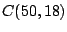and 17!! because we are choosing 18 cards from 50 to be dealt to the other nine players, and there are 17!! ways to partition the 18 cards into nine hands of two cards each. Then set aside the remaining two aces to go into another hand. For the other eight hands, we are choosing 16 cards from 48 and partitioning the 16 cards in 15!! ways to form completions.

This gives us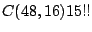completions to player semideals with another pair of pocket aces. Dividing the second number by the first gives us a probability of 9/1,225 that someone else also has been dealt pocket aces. Expressed as a decimal, the probability is about .00735, and expressed as an Egyptian fraction, the probability is about 1/136.

Therefore, if you are dealt pocket aces, the odds against another player also having pocket aces is about 135-to-1.

Home | Publications | Preprints | MITACS | Poker Digest | Poker Computations | Feedback
website by the Centre for Systems Science
last updated 4 December 2002